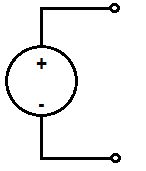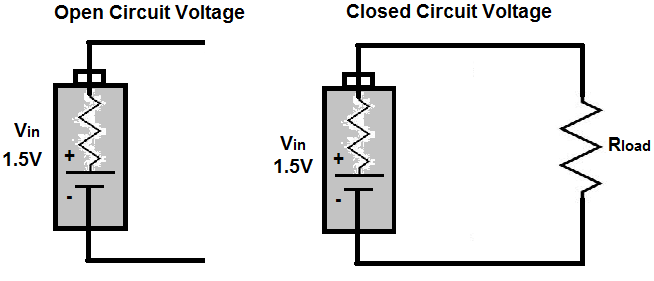﻿ What is Open Circuit Voltage? ﻿# What is Open Circuit Voltage?Open circuit voltage (or potential) is voltage which is not connected to any load in a circuit.

See the two examples below to see the difference between open circuit voltage with a voltage source which is in a closed circuit and connected to a load.As you can see, open circuit voltage is disconnected and does not form a complete circuit. This is why it is called open. It is open and not connected to a form a complete electrical path.

### Open Circuit Voltage Represents a Voltage Source's Full Voltage

Because it doesn't drop any voltage across a load, as what would happen when it is connected to a load, a voltage source's open circuit voltage represents its full voltage value, since the voltage doesn't share any of its voltage with a load.

A prime example below are these two battery circuits:The battery to the left is unconnected to any load. Assuming that this 1.5-volt battery is new and has full operational life of 1.5 volts, it will measure the full 1.5 volts across it when we take a voltmeter and place the meter's probes across its terminals.

The battery to the right, now, is connected to a load. Again, assuming that this battery has full life of 1.5 volts, it will only retain 0.17V of the 1.5V. The remaining voltage will be dropped across the 8Ω resistor. This is calculated by using ohm's law, I=V/R. Since there is 9Ω in the circuit and the voltage source is 1.5V, the current going through the circuit is I= 1.5V/9Ω= 0.17A. We now use a revised version of ohm's law, V=IR, to calculate the voltages across the battery and the resistor. Since the battery has an internal resistance of 1Ω, it drops V= 0.17A * 1Ω= 0.17V. The resistor has a voltage drop of V= 0.17A * 8Ω= 1.36V.

### Open Circuit Voltage Is Real Voltage

There is a misconception many times that because a voltage source isn't connected to a circuit or load, the voltage doesn't really exist or its power doesn't go into effect until it is connected to a closed circuit path. However, this is erroneous.

A voltage source does not need to be connected to anything for voltage to exist. Voltage unconnected is real voltage. Even if a voltage source is unconnected and not attached to any load, the potential power still exists. A prime example of this is the voltage which comes out of a wall outlet. The standard value for the US is 120VAC. Even when nothing is plugged into the wall outlet, the potential across the power terminal's power supply and ground of the outlet will still measure 120VAC with nothing connected. The hazard of getting shocked from a wall outlet is still there.

This misconception may stem from the fact that current can only flow when it is in a closed circuit. However, voltage is different. While current can only exist when a circuit is closed, voltage always has potential.

Related Resources

HTML Comment Box is loading comments...

﻿